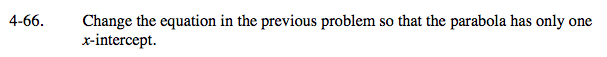### Home > A2C > Chapter 4 > Lesson 4.2.1 > Problem4-66

4-66.Remember that the parabola's vertex was

$\left(-\frac{3}{4}, -\frac{1}{8}\right)$

Remember that the only way for a parabola to have only one x-intercept is to have the vertex touching the x-axis.

To make it so the equation only has one x-intercept, you must make the vertex's y value equal 0.

$\textit{y}=2\textit{x}^{2}+3\textit{x}+\frac{9}{8}$